# Rounding

The following numbers round to the thousandth:

-54.3191:  -54.319
-86.6571:  -86.657
66.2407:  66.241
-72.3526:  -72.353
25.4285:  25.429
-93.3033:  -93.303
46.1996:  46.2
36.542:  36.542
94.6223:  94.622
-54.1494:  -54.149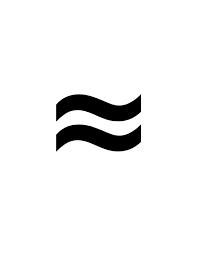Did you find an error or inaccuracy? Feel free to write us. Thank you!Tips to related online calculators
Do you want to round the number?

## Related math problems and questions:

• Round it0.728 round to units, tenths, hundredths.
• Sum and roundingI know two numbers whose sum is 20. When they each round and then added together I get the 30. What are this numbers?
• Rounding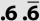Double round number 727, first to tens, then to hundreds. (double rounding)
• Rounding 2Round the following negative number: -143.021
• Round 9Round number 0.2375 TO 2 SIGNIFICANT FIGURES
• Video gameNicole is playing a video game where each round lasts 7/12 of an hour. She has scheduled 3 3/4 hours to play the game. How many rounds can Nicole play?
• Valid numberRound the 453874528 on 2 significant numbers.
• EstimateEstimate the answer by rounding each fraction to the nearest whole number and then subtracting. 9/15 - 2 7/8
• The equator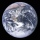Calculate the length of the equator. Calculate a radius of 6378 km. Round to the nearest thousand. - Procedure and calculation
• A merry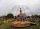A merry go round can hold 36 people on each 5 minute ride. How many people would have received a ride on the merry go round in an hour?
• Brian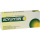Brian divides 80 by 7. Between what two whole numbers is his answer?
• How many 14How many 1/2 cup serving are in a package of cheese that contains 5 1/4 cups altogether?
• WillieWillie collected 6 2/3 pails of water. How many big containers can she fill? Does each container contain 2 2/9 of a pail?
• NumbersDetermine the number of all positive integers less than 4183444 if each is divisible by 29, 7, 17. What is its sum?
• How manyHow many integers are greater than 547/3 and less than 931/4?
• RemainderWhat is the remainder of the division of natural numbers 293 and 7?
• Yogurt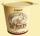The board is 35 yogurts. How many boards of yogurts must be ordered for 886 people in the canteen?